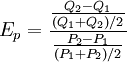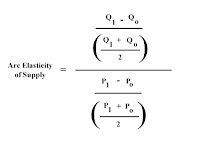3/03/2009

Arc Elasticity

Let us calculate an easy PED:

Price(OLD)=10
Price(NEW)=9
QDemand(OLD)=110
QDemand(NEW)=150

PED formula: (%change in quantity demanded)\(%change in price)

the change in quantity demanded is 0.3636 (trust me, I use calculator) and the change in price is -0.1

PED = (0.3636)/(-0.1) = -3.636

ok, now lets do it opposite way:
Price(NEW)=10
Price(OLD)=9
QDemand(NEW)=110
QDemand(OLD)=150

the change in quantity demanded is -0.2667 (calculator is love) and the change in price is 0.1111

PED = (-0.2667)/(0.1111) = -2.4005

Wait a second, elasticities differ, but the data is the same!
When calculating a price elasticity, we drop the negative sign, so our final value is 3.636. Obviously 3.6 is a lot different from 2.4, so we see that this way of measuring price elasticity is quite sensitive to which of your two points you choose as your new point, and which you choose as your old point. Arc elasticities are a way of removing this problem.

Ok, using arc elasticity formula, we would calculate the percentage change in Quantity Demand the following way:

[[QDemand(NEW) - QDemand(OLD)] / [QDemand(OLD) + QDemand(NEW)]]*2

This formula takes an average of the old quantity demanded and the new quantity demanded on the denominator. By doing so, we will get the same answer (in absolute terms) by choosing \$9 as old and \$10 as new, as we would choosing \$10 as old and \$9 as new.

The same transformations are happening with the price changes calculations when using arc elsticity:
[[Price(NEW) - Price(OLD)] / [Price(OLD) + Price(NEW)]] *2]

Therefore the formula of Arc Price Elasticity of Demand is:
[[Price(NEW) - Price(OLD)] / [Price(OLD) + Price(NEW)]] *2] over [[Price(NEW) - Price(OLD)] / [Price(OLD) + Price(NEW)]] *2]

or, to make you imagine that, I found a fraction pic:You can also count Arc Price Elasticity of Supply, Arc Income Elasticity of Demand, Arc Cross Elasticity of Demand of good X.

APES = [[QSupply(NEW) - QSupply(OLD)] / [QSupply(OLD) + QSupply(NEW)]] *2] over [[Price(NEW) - Price(OLD)] / [Price(OLD) + Price(NEW)]] *2]

or_________

AIED = [[QDemand(NEW) - QDemand(OLD)] / [QDemand(OLD) + QDemand(NEW)]] *2] over [[Income(NEW) - Income(OLD)] / [Income(OLD) + Income(NEW)]] *2]

AXED = [[QDemand(NEW) - QDemand(OLD)] / [QDemand(OLD) + QDemand(NEW)]] *2] over [[Price(NEW) - Price(OLD)] / [Price(OLD) + Price(NEW)]] *2]

Don't worry, it all looks scary and enormous just because I'm typing on my laptop and obviously I cannot use fractions - it would look much clearer and very nice!
If you want, I can explain it to you at school at any time, just ask (:

Source: